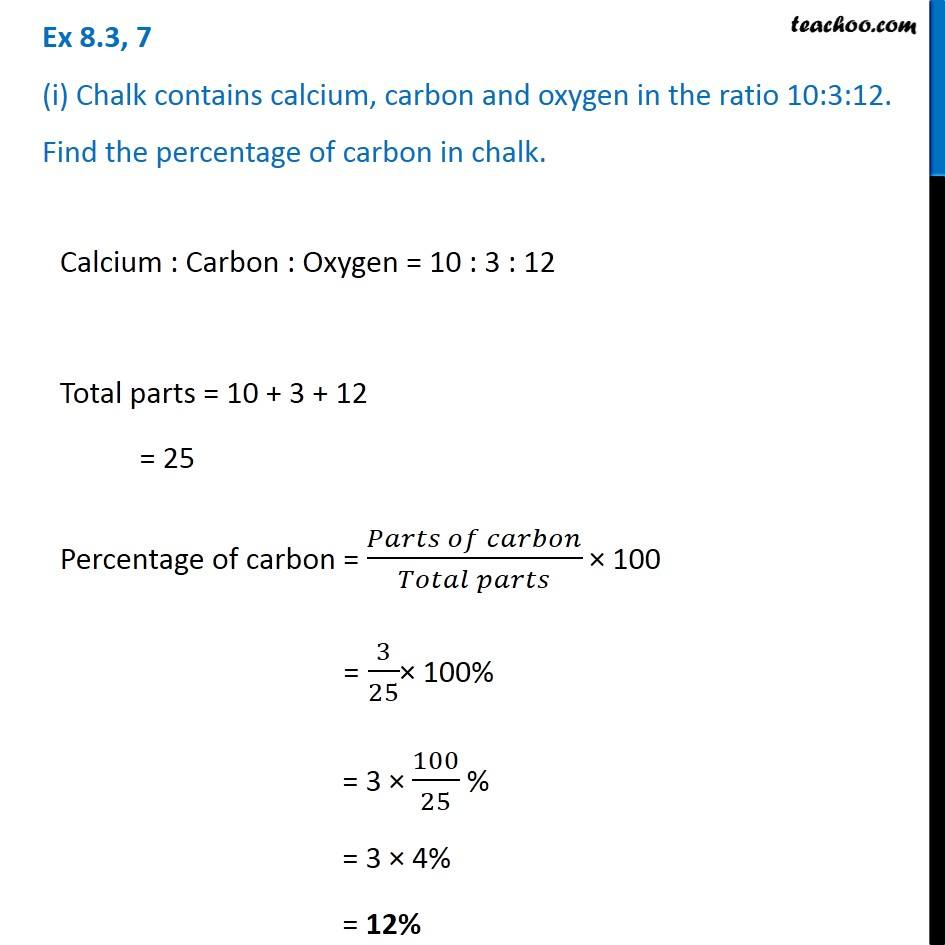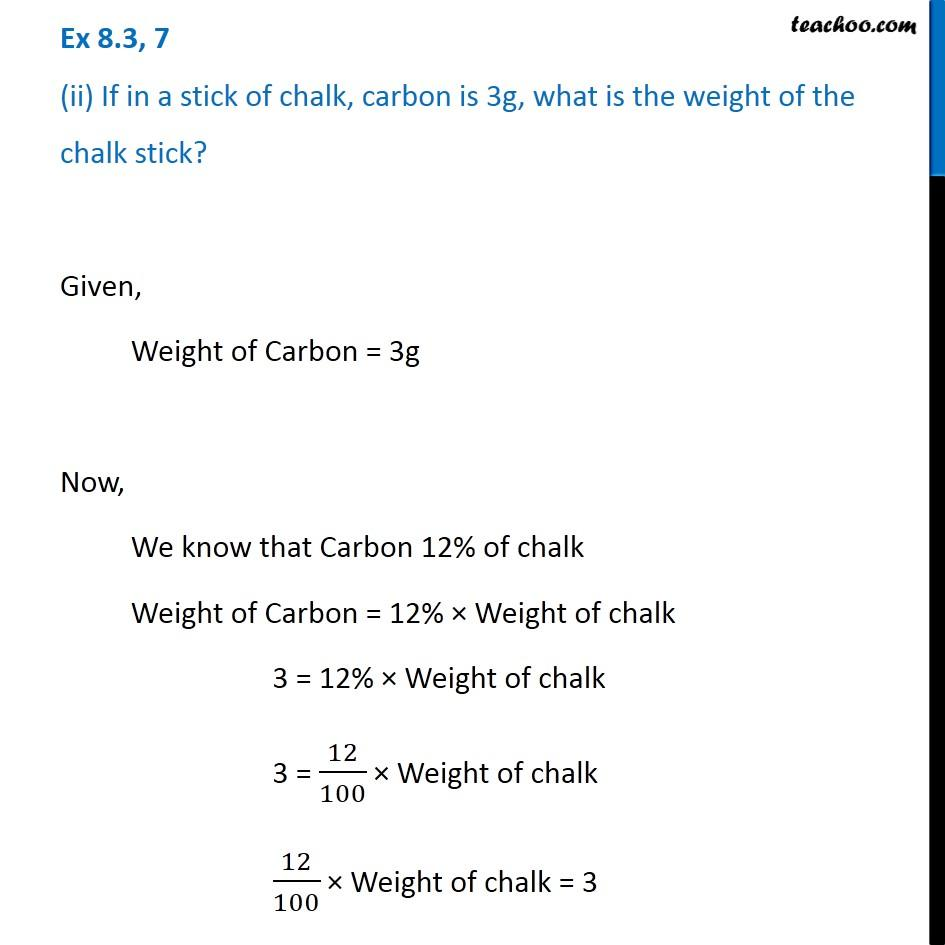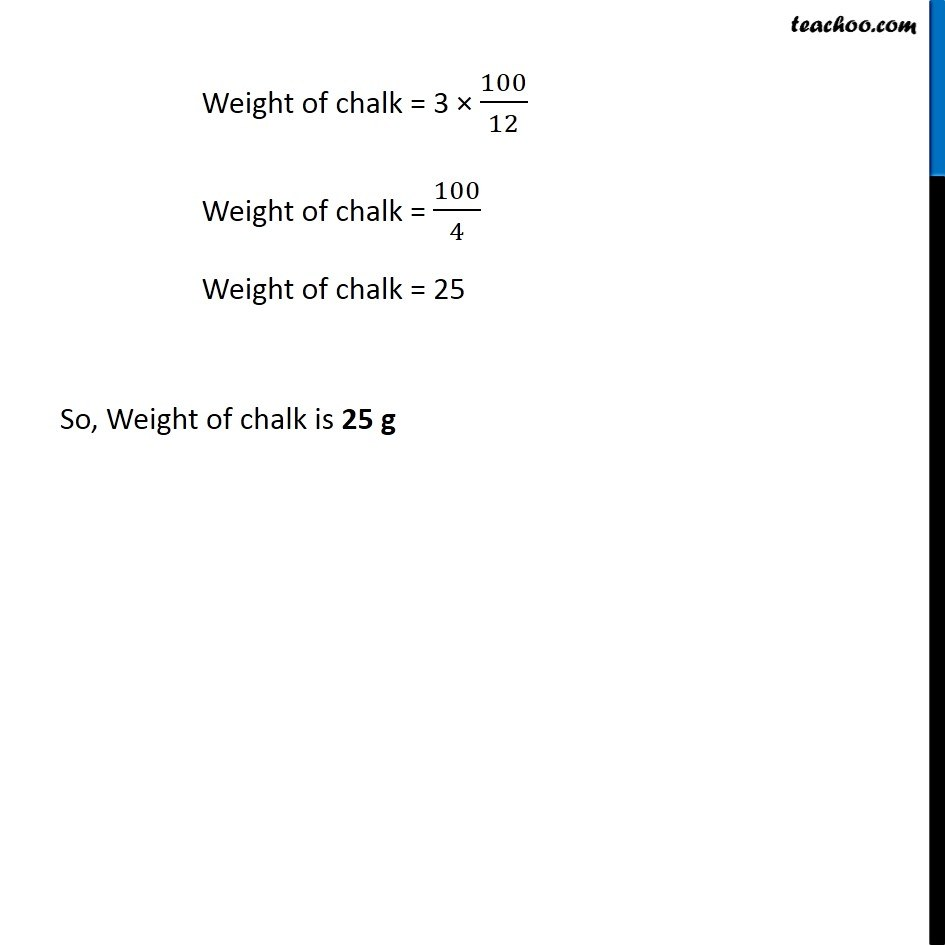Ex 8.3

Chapter 8 Class 7 Comparing Quantities
Serial order wiseGet live Maths 1-on-1 Classs - Class 6 to 12

### Transcript

Ex 8.3, 7 (i) Chalk contains calcium, carbon and oxygen in the ratio 10:3:12. Find the percentage of carbon in chalk. Calcium : Carbon : Oxygen = 10 : 3 : 12 Total parts = 10 + 3 + 12 = 25 Percentage of carbon = (𝑃𝑎𝑟𝑡𝑠 𝑜𝑓 𝑐𝑎𝑟𝑏𝑜𝑛)/(𝑇𝑜𝑡𝑎𝑙 𝑝𝑎𝑟𝑡𝑠) × 100 = 3/25× 100% = 3 × 100/25 % = 3 × 4% = 12% Ex 8.3, 7 (ii) If in a stick of chalk, carbon is 3g, what is the weight of the chalk stick? Given, Weight of Carbon = 3g Now, We know that Carbon 12% of chalk Weight of Carbon = 12% × Weight of chalk 3 = 12% × Weight of chalk 3 = 12/100 × Weight of chalk 12/100 × Weight of chalk = 3 Weight of chalk = 3 × 100/12 Weight of chalk = 100/4 Weight of chalk = 25 So, Weight of chalk is 25 g Weight of chalk = 3 × 100/12 Weight of chalk = 100/4 Weight of chalk = 25 So, Weight of chalk is 25 g